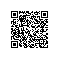# Linux脚本中带有小数点的数值比较大小

 1 2 3 4 5 6 7 8 9 10 11 a=1.2 b=1.1   如果直接用 if [ $a -gt $b ];then echo "$a >$b" fi 或者 if [ $a > $b ];then echo "$a >$b" fi

[: 1.2: integer expression expected

shell的 test 对于数值的判断都是基于整数的,没办法对带有小数的数值直接进行对比。

1、[[   ]]

 1 [[ $a > $b ]] &&  echo "$a >$b"

2、expr方法

 1 2 3 4 5 6 7 a=1.2 b=1.1 if [ expr $a \> $b -eq 0 ];then echo "$a <$b" else echo "$a >$b" fi

3、bc计算（推荐使用）

 1 2 3 4 5 6 7 8 a=1.0 b=1.1   if [ $(echo "$a > $b"|bc) -eq 0 ];then echo "$a < $b" else echo "$a > $b" fi 大于返回1，小于返回0 位数不一样的有小数的数字，判断是否相等：  1 2 3 4 5 6 7 8 a=1.0 b=1.0000 if [$(echo "$a ==$b"|bc) -eq 0 ];then echo "$a !=$b" else echo "$a =$b" fi

4、awk

 1 awk -v num1=6.6 -v num2=5.5 'BEGIN{print(num1>num2)?"0":"1"}'使用钉钉扫一扫加入圈子
+ 订阅